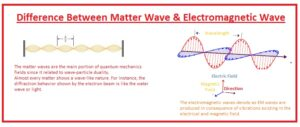Hi, readers welcome to the new post. In this post, we will have a detailed look at Difference Between Matter Wave & Electromagnetic Wave.  Wave defines as disturbance created in the medium. In different situations wave defined through wave equation For the creation of physical waves, there is the use of 2 quantities in that particle place where the wave has to create.

There is a type of wave called periodic wave here waves show fluctuation about the position of rest. The common type of waves is 3 through which electromagnetic and matter waves are. The basic comparison is such that in electromagnetic waves there is combination o electrical and magnetic fields while in matter waves it not exist. In this post, we will cover their basics with detail. So let’s get started

## Difference Between Matter Wave & Electromagnetic Wave

Matter Wave

• The matter waves are the main portion of quantum mechanics fields since it related to wave-particle duality.
• Almost every matter shows a wave-like nature. For instance, the diffraction behavior shown by the electron beam is like the water wave or light.
• In certain conditions, the wavelength is very less to show the physical results at different factors.
• The wave-like features shown by any matter is given by Louise de Broglie. That called de Broglie hypotheses.
• So these waves also called de Broglie waves While the Broglie wavelength is the wavelength that is related to high weight particles and has a relation with the plank constant and momentum.

λ=h/P

#### Electromagnetic Wave

• The electromagnetic waves denote as EM waves are produced in consequence of vibrations existing in the electrical and magnetic field.
• In a basic way, electromagnetic waves are comprised of electric and magnetic fields in oscillation.
• These waves are created when there is a connection among the electrical field and magnetic field. So this wave known as electromagnetic waves.
• The electrical fields and magnetic fields of in these waves at ninety degrees to each other.
• The traveling wave of these waves are 3 x 10Λ8 meters per second in space. There is no deflection shown by these fields is not from electrical and magnetic fields.
• Since the waves showed diffraction behavior. These waves can pass from any material like solid, air,.
• There is no need of any certain medium for these waves.What is Electromagnetic Waves

• There is a need of electric and magnetic fields for these waves.
• These waves can pass through the vacuum.
• Its example is UV, radio wave.
• Their speed of movement is close to the speed of light.
• Its equation is λ=c/v

What is Matter Wave

• There is no need of a certain type of field for these waves.
• These waves can pass through wave sine.
• An example of this wave is a beam of electrons.
• Its speed of movement is less than the speed of light.
• λ=h/P is its equation.

That is a detailed post about the Difference Between Matter Wave & Electromagnetic Wave if you have any further query ask in comments. Thanks for reading. have a nice day.